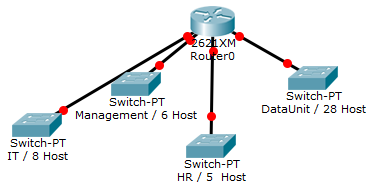# Subnetting with Variable-Length Subnet Mask (VLSM)

In this tutorial, we will go through steps of subnetting a network address using multiple subnet masks. If you’re looking for classful subnetting, you checkout my other tutorial here. After the detailed steps, we will go through two completely solved example and three more unsolved examples for you to solve. Grab a pencil and a piece of paper and let’s go.

To start with, we will assume that you have a specific number of subnets with a specific number of hosts in each subnet. What we will do in the process of subnetting is identifying the subnet address, subnet mask, subnet broadcast address, range of available host addresses for each subnet.

Subnetting generally means using some bits of the host address to generate subnet address.

Subnetting Steps:

Step 1: Choosing the Network Address

If you were given a specific network address to use in subnetting, jump to step 2. If it is up to you to choose the network address and IP address class, you may select it based on the following steps:

1. For each subnet calculate a BlockSize.
2. The BlockSize is calculated by taking the No. of Hosts+2 and rounding it to the next power of 2 (ex: 2, 4, 8, 16, 32, 64..etc which is actually 21, 22, 23, 24, 25,..etc). When we say “round to the next”, it means that 17 is rounded to 32 and 5 is rounded to 8. The result of rounding is the BlockSize. Now we have the BlockSize for each subnet.

Note: We put +2 because the subnet address and the broadcast address cannot be used by hosts.

1. Add all BlockSize for all subnets. If the sum is 256 or less, you can go for Class C address. If the result is higher than 28(which equals 256) but less than 216 (which equals 65,536), go for Class B. If the result is more than 216, go for Class A.
2. Since subnetting mostly happens for LANs, the addresses that we will use are in the Private ranges of each class as shown in the table:
 Address Class Private Range of IP addresses C 192.168.0.0 – 192.168.255.255 B 172.16.0.0 – 172.16.255.255 A 10.0.0.0 – 10.255.255.255

For the sake of simplicity, we usually choose 192.168.0.0 for Class C, and 172.16.0.0 for Class B.

Step 2: Choosing the Subnet Mask for Each Subnet

The calculation is really simple. Using the BlockSize that you have calculated in Step 1, you can find the number of bits required for host address after subnetting (Lets call it HBits) by the following calculation:

BlockSize=2HBits

This means that only HBits number of bits will be used for host addresses and the rest of the bits will be used for subnet addresses.

From that, you can easily calculate the number of bits used for subnet address (lets call it NBits) by:

NBits=32-HBits

Now you can produce the subnet mask by starting (from the left) with NBits ones and HBits zeros afterwards.

For example, if NBits=20 and HBits=12

1111 1111.1111 1111.1111 0000.0000 0000

255.255.240.0

Repeat this step for each subnet.

Step 3: Filling-up the Table

The first thing to do is to re-order subnets in according to their BlockSize in descending order. This is extremely important.

The first subnet address is usually the main network address. Next subnet address can be easily calculated by adding 1 to the network part of the previous subnet address. Remember to do the addition in binary, so that carry bits can be pushed to the next octet (8-bits) on the left.

For example:

The first subnet 192.168.0.0 and the subnet mask is 255.255.255.240. In binary:

 Subnet Mask for Subnet 0 (255.255.255.240) 1111 1111 . 1111 1111 . 1111 1111 . 1111       0000 Network (and also the address of Subnet 0, 192.168.0.0) Host 1100 0000 . 1010 1000 . 0000 0000 . 0000 0000 Subnet Mask for Subnet 1 (255.255.255.248) 1111 1111 . 1111 1111 . 1111 1111 . 1111       1000 Subnet 1 (192.168.0.16) Host 1100 0000 . 1010 1000 . 0000 0000 . 0001  0 000 Subnet 2 (192.168.0.24) Host 1100 0000 . 1010 1000 . 0000 0000 . 0001   1 000 Subnet 3, 4, 5,….

You can see in the table that the subnet addresses are calculated by adding 1 to the network-part of the previous subnet address.

1. Subnet mask for each subnet was earlier calculated in Step 2.
2. Broadcast address of each subnet is the last address before the next subnet. In follow-up of the previous example in 1, if subnet 0 address is 192.168.0.0 and subnet 1 is 192.168.0.16, then the broadcast address of subnet 0 is 192.168.0.15.
3. Usable host range is simply all addresses between the subnet address and the broadcast address. Again following-up on the previous example in 1, and 2, for subnet 0 the subnet address is 192.168.0.0 and broadcast address 192.168.0.15, then the usable host range is 192.168.0.1-192.168.0.14

Example 1:

You have been assigned the duty of subnetting a network for an organization’s branch office. The headquarter’s IT manager said that you need to use the network address 192.168.12.0 with the default subnet mask (255.255.255.0). The number of hosts is shown in the diagram below.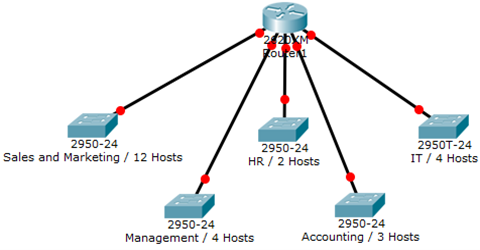Solution:

Since the main network address is already selected by the main office, we will directly start calculating the block size

HiHost=12

BlockSize= 12+2 rounded-up to the nearest power of 2.

BlockSize=16

Now we calculate the resulting subnet mask:

2HBits=16  ® 2HBits=24 ® HBits=4

NBits=32-4=28

Thus,

Subnet Mask is 1111 1111.1111 1111.1111 1111.1111 0000 ® 255.255.255.240

Now we start calculating subnets

 Subnet Mask (255.255.255.240) 1111 1111 . 1111 1111 . 1111 1111 . 1111       0000 Network (and also the address of subnet 0, 192.168.12.0) Host 1100 0000 . 1010 1000 . 0000 1100 . 0000 0000 Subnet 1 (192.168.12.16) 1100 0000 . 1010 1000 . 0000 1100 . 0001 0000 Subnet 2 (192.168.12.32) 1100 0000 . 1010 1000 . 0000 1100 . 0010 0000 Subnet 3 (192.168.12.48) 1100 0000 . 1010 1000 . 0000 1100 . 0011 0000 Subnet 4 (192.168.12.64) 1100 0000 . 1010 1000 . 0000 1100 . 0100 0000

You can clearly notice the gradual increment in the subnet address within its first part on the right while host bits remain zero all the time.

Now we start filling the table:

 S/N No S/N Address S/N Mask Broadcast Address Range of Host Addresses 0 192.168.12.0 255.255.255.240 192.168.12.15 192.168.12.1 – 192.168.12.14 1 192.168.12.16 255.255.255.240 192.168.12.31 192.168.12.17 – 192.168.12.30 2 192.168.12.32 255.255.255.240 192.168.12.47 192.168.12.33 – 192.168.12.46 3 192.168.12.48 255.255.255.240 192.168.12.63 192.168.12.49 – 192.168.12.62 4 192.168.12.64 255.255.255.240 192.168.12.79 192.168.12.65 – 192.168.12.78

Example 2:

You have been assigned the duty of subnetting a network for an organization. The choice of class and network address is up to you. Take into consideration the host numbers mentioned in the diagram below.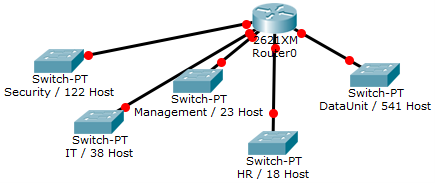Solution:

In order to make a proper network address and class choice, let us calculate the BlockSize first.

HiHost=541 ® BlockSize=541+2 rounded-up to the nearest power of two= 1024

Since the BlockSize is higher than 28 and lower than 216, we will choose class B

Let the network address be 172.16.0.0 and the default subnet mask be 255.255.0.0

Now we will calculate the subnet mask after subnetting,

BlockSize=2HBits ® 1024=2HBits ® 210=2HBits ® HBits=10

NBits=32-10=22

1111 1111.1111 1111.1111 1100.0000 0000 ® 255.255.252.0

Now we start calculating subnets

 Subnet Mask (255.255.252.0) 1111 1111 . 1111 1111 . 1111     11 00 . 0000       0000 Network (and also the address of subnet 0, 172.16.0.0) Host 1010 1100 . 0001 0000 . 0000     00 00 . 0000 0000 Subnet 1 (172.16.4.0) 1010 1100 . 0001 0000 . 0000     01 00 . 0000 0000 Subnet 2 (172.16.8.0) 1010 1100 . 0001 0000 . 0000    10 00 . 0000 0000 Subnet 3 (172.16.12.0) 1010 1100 . 0001 0000 . 0000    11 00 . 0000 0000 Subnet 4 (172.16.16.0) 1010 1100 . 0001 0000 0001    00 00 . 0000 0000

You can clearly notice the gradual increment in the subnet address within its first part on the right while host bits remain zero all the time.

Now we start filling the table:

 S/N No S/N Address S/N Mask Broadcast Address Range of Host Addresses 0 172.16.0.0 255.255.252.0 172.16.3.255 172.16.0.1 – 172.16.3.254 1 172.16.4.0 255.255.252.0 172.16.7.255 172.16.4.1 – 172.16.7.254 2 172.16.8.0 255.255.252.0 172.16.11.255 172.16.8.1 – 172.16.11.254 3 172.16.12.0 255.255.252.0 172.16.15.255 172.16.12.1 – 172.16.15.254 4 172.16.16.0 255.255.252.0 172.16.19.255 172.16.16.1 – 172.16.19.254

Unsolved Examples:

1. You have been assigned the duty of subnetting a network for an organization. The choice of class and network address is up to you. Take into consideration the host numbers mentioned in the diagram below.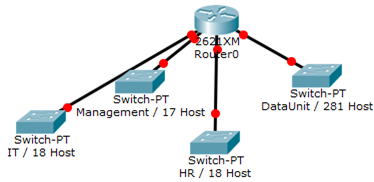1. You have been assigned the duty of subnetting a network for an organization’s branch office. The headquarter’s IT manager said that you need to use the network address 172.18.0.0 with the default subnet mask (255.255.0.0). The number of hosts is shown in the diagram below.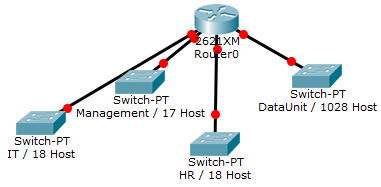1. You have been assigned the duty of subnetting a network for an organization. The choice of class and network address is up to you. Take into consideration the host numbers mentioned in the diagram below.